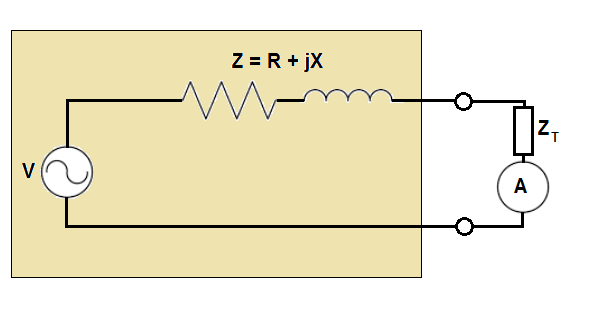# Black Box Impedance SleuthingSuppose there is a box containing an ideal sinusoidal AC voltage source (RMS magnitude of $V$) in series with a resistance $R$ and an inductive reactance $X$ (for a total complex impedance $Z = R + jX)$. The circuit is incomplete, and a pair of terminals are brought outside the box. Aside from the availability of the terminals, the box is closed and its contents are inaccessible.

You want to find out the values of the resistance and inductive reactance, so you devise a clever strategy to determine them. You take three measurements, each time connecting an ideal AC ammeter in series with a test impedance $Z_T$ and connecting the series combination across the box terminals.

For the first measurement, $Z_T$ is a perfect short-circuit with zero impedance, and the measured RMS current magnitude is $\Large{\frac{V}{\sqrt{\alpha}}}$.

For the second measurement, $Z_T$ is a $1 \Omega$ resistance, and the measured RMS current magnitude is $\Large{\frac{V}{\sqrt{\alpha+5}}}$.

For the third measurement, $Z_T$ is a $1 \Omega$ inductive reactance, and the measured RMS current magnitude is $\Large{\frac{V}{\sqrt{\alpha+9}}}$.

What is the value of $R + X$?

Note: In electrical engineering, the letter $''j''$ is commonly used to denote $\sqrt{-1}$, since the letter $''i''$ is commonly used to represent current.

×

Problem Loading...

Note Loading...

Set Loading...